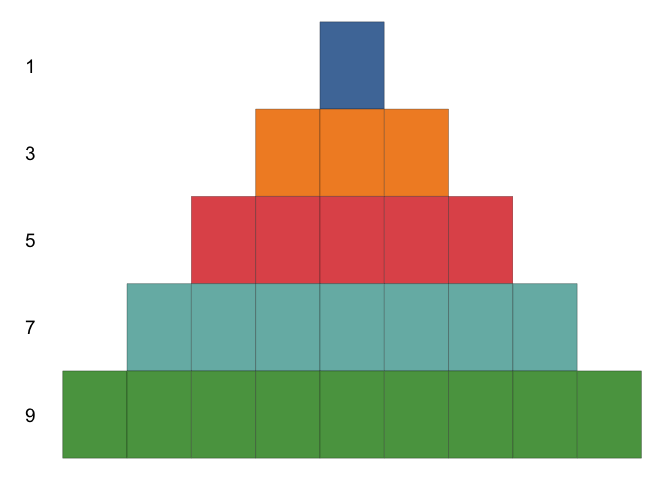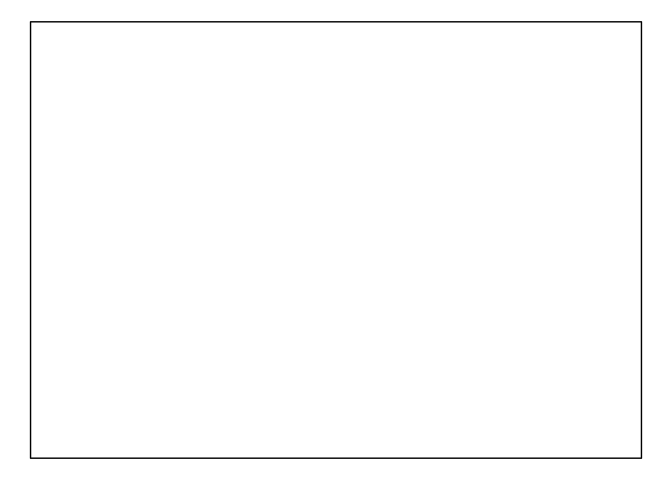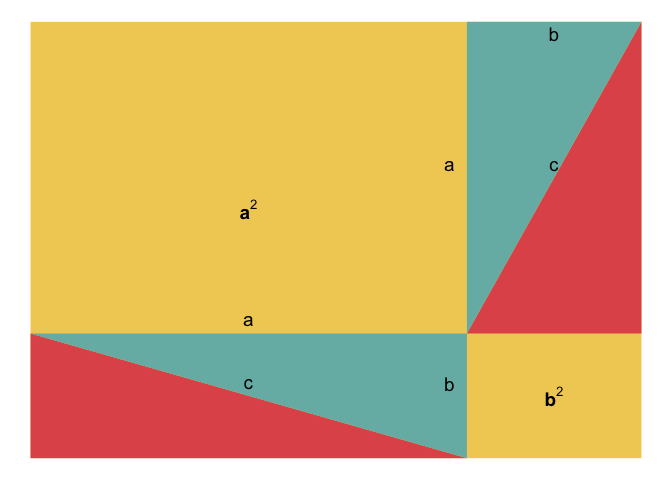[This article was first published on R on Notes of a Dabbler, and kindly contributed to R-bloggers]. (You can report issue about the content on this page here)
Want to share your content on R-bloggers? click here if you have a blog, or here if you don't.

I recently watched the 2 part workshop (part 1, part 2) on ggplot2 and extensions given by Thomas Lin Pedersen. First of, it was really nice of Thomas to give the close to 4 hour workshop for the benefit of the community. I personally learnt a lot from it. I wanted to try out gganimate extension that was covered during the workshop.

There are several resources on the web that show animations/illustrations of proofs of mathematical identities and theorems without words (or close to it). I wanted to take a few of those examples and use gganimate to recreate the illustration. This was a fun way for me to try out gganimate.

## Example 1:

This example is taken from AoPS Online and the result is that sum of first $$n$$ odd numbers equals $$n^2$$. $1 + 3 + 5 + \ldots + (2n – 1) = n^2$ The gganimate version of the proof (using the method in AoPS Online) is shown below (R code, html file)## Example 2:

This example is also taken from AoPS Online and the result is:

$1^3 + 2^3 + \ldots + (n-1)^3 + n^3 = (1 + 2 + \ldots + n)^2$ The gganimate version of the proof (using the method in AoPS Online) is shown below ( R code, html file):## Example 3

This example from AoPS Online illustrates the result

$\frac{1}{2^2} + \frac{1}{2^4} + \frac{1}{2^6} + \frac{1}{2^8} + \ldots = \frac{1}{3}$ The gganimate version of the proof (using the method in AoPS Online) is shown below ( R code, html file):## Example 4

According to Pythagoras theorem, $a^2 + b^2 = c^2$ where $$a$$, $$b$$, $$c$$ are sides of a right angled triangle (with $$c$$ being the side opposite $$90^o$$ angle)

There was an illustration of the proof of pythogoras theorem in a video from echalk.

The gganimate version of the proof is shown below ( R code, html file)In summary, it was great to use gganimate for these animations since it does all the magic with making transitions work nicely.